Test: Criteria for Similar Triangles

# Test: Criteria for Similar Triangles

Test Description

## 10 Questions MCQ Test Mathematics (Maths) Class 10 | Test: Criteria for Similar Triangles

Test: Criteria for Similar Triangles for Class 10 2022 is part of Mathematics (Maths) Class 10 preparation. The Test: Criteria for Similar Triangles questions and answers have been prepared according to the Class 10 exam syllabus.The Test: Criteria for Similar Triangles MCQs are made for Class 10 2022 Exam. Find important definitions, questions, notes, meanings, examples, exercises, MCQs and online tests for Test: Criteria for Similar Triangles below.
Solutions of Test: Criteria for Similar Triangles questions in English are available as part of our Mathematics (Maths) Class 10 for Class 10 & Test: Criteria for Similar Triangles solutions in Hindi for Mathematics (Maths) Class 10 course. Download more important topics, notes, lectures and mock test series for Class 10 Exam by signing up for free. Attempt Test: Criteria for Similar Triangles | 10 questions in 10 minutes | Mock test for Class 10 preparation | Free important questions MCQ to study Mathematics (Maths) Class 10 for Class 10 Exam | Download free PDF with solutions
 1 Crore+ students have signed up on EduRev. Have you?
Test: Criteria for Similar Triangles - Question 1

### If ΔDEF ~ ΔABC and DE = AB, what is the relation between the two triangles?​

Detailed Solution for Test: Criteria for Similar Triangles - Question 1

Since DE=AB means that there ratio is 1 which means corresponding sides are equal.Two triangles are said to be similar if their corresponding angles are congruent and the corresponding sides are in proportion . In other words, similar triangles are the same shape, but not necessarily the same size. The triangles are congruent if, in addition to this, their corresponding sides are of equal length.So they are congruent.

Test: Criteria for Similar Triangles - Question 2

### In the following figure, find the value of x.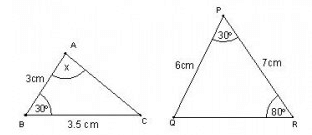​

Detailed Solution for Test: Criteria for Similar Triangles - Question 2

The triangles are similar by SAS criterion, So we have, Angle A+Angle B + Angle C=180
x=180-30-80=70 degrees

Test: Criteria for Similar Triangles - Question 3

### In the figure given below, if DE || BC, then x equals :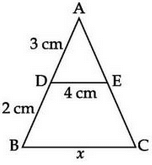Detailed Solution for Test: Criteria for Similar Triangles - Question 3

We have Angle ADE= Angle ABC
And ANGLE A is common, So by AA criterion of similarity the two triangles are similar so AD/AB=DE/BC
x=5*4/3=6.7

Test: Criteria for Similar Triangles - Question 4

Given that ΔABC ~ ΔDEF and AB = 2cm , BC = 3cm, DE = 4cm, EF = 6cm , If DF = 8cm, then AC = ?​

Detailed Solution for Test: Criteria for Similar Triangles - Question 4

We have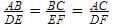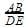=2/4=½
So,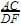AC=8/2=4cm

Test: Criteria for Similar Triangles - Question 5

If ΔPQR ~ ΔXYZ, ∠Q = 50°, ∠R = 70° then ∠X is equal to :​

Test: Criteria for Similar Triangles - Question 6

Given that ΔABC ~ ΔDEF ∠A = 50°, ∠C = 35° ∠E = ?

Test: Criteria for Similar Triangles - Question 7

In the given figure perpendiculars are dropped on the common base BD of the given two triangles. AE = 2cm, CF = 3cm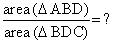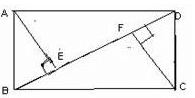Detailed Solution for Test: Criteria for Similar Triangles - Question 7

Area of triangle = 1/2 *base*height
Area of ABD=1/2 *BD*2
Area of  BDC=1/2*BD*3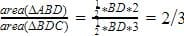Test: Criteria for Similar Triangles - Question 8

In the given figure ΔABC ~ ΔBDC = 90° each. Choose the correct similarity from the given choices.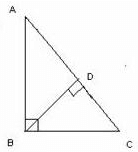Detailed Solution for Test: Criteria for Similar Triangles - Question 8

We have Angle C common and Angle B = Angle D
So in similarity we write the name of the triangle in such an order in which the corresponding alphabets denote equal angles . this means that ΔABC ~ ΔBDC that says Angle A = Angle B, Angle B = Angle D, and Angle C = Angle C

Test: Criteria for Similar Triangles - Question 9

ΔABC ~ ΔDEF Perimeter (ΔABC) = 15 cm, Perimeter (DEF) = 25 cm.If AB = 6 cm, then find DE.

Detailed Solution for Test: Criteria for Similar Triangles - Question 9

Ratios of perimeter of similar triangles are equal to the ratio of their sides.
So Perimeter of ABC/perimeter of DEF=15/25=⅗
AB/DE=⅗
DE=6*5/3=10

Test: Criteria for Similar Triangles - Question 10

In the given figure use the similarity of the given triangles to find the value of BD,

ΔABC ~ ΔBDC,  ∠BDC = ∠ABC = 90°, AB = 3, BC = 4,AD = 2, BD = ?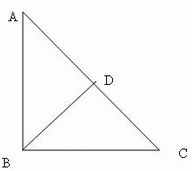Detailed Solution for Test: Criteria for Similar Triangles - Question 10

Solution :

The correct option is Option C.

∆ ABC ~ ∆ BDC = AD/AB = BD/BC = ⅔ = BD/4 = BD = 8/3

## Mathematics (Maths) Class 10

53 videos|369 docs|138 tests
 Use Code STAYHOME200 and get INR 200 additional OFF Use Coupon Code
Information about Test: Criteria for Similar Triangles Page
In this test you can find the Exam questions for Test: Criteria for Similar Triangles solved & explained in the simplest way possible. Besides giving Questions and answers for Test: Criteria for Similar Triangles, EduRev gives you an ample number of Online tests for practice

## Mathematics (Maths) Class 10

53 videos|369 docs|138 tests